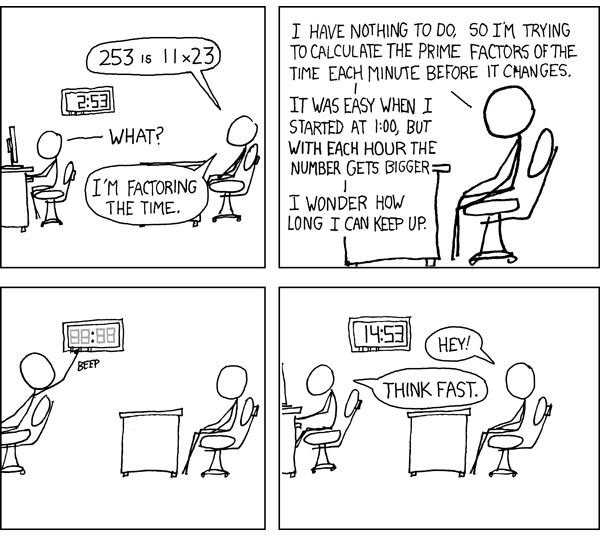# What is Prime Factorization? Explanation, Examples & Practice Questions

## What is Prime Factorization?

One of the basic concepts of mathematics is expressing numbers in terms of smaller numbers, whether by addition (seven is the sum of three and four), multiplication (12 is the product of three and four), or exponentiation (81 is three to the fourth power). We often find it useful to break a number down into smaller numbers. Given a number, we call any number that divides evenly into it one of its factors, where dividing evenly means there is no remainder. For example, the factors of 12 are 1, 2, 3, 4, 6 and 12, because 12 can be divided by each of these with no remainder.

So what is prime factorization? Recall that a prime number is any whole number (that is, a positive integer) that can only be divided by itself and one; every whole number that is not prime is composite, with the exception of one itself, which is neither prime nor composite. The prime factors of a number are just those factors that happen to be prime; the fundamental theorem of arithmetic states that every positive integer can be uniquely factored into a set of prime numbers, which we call the prime factorization.

## Examples

For example, let’s find the prime factorization of the number 12; we cando this using a factor tree or by repeated division. Twelve is the product of three and four, three is prime, four is the product of two and two, and two is prime. Thus, we see that 12 = 2 x 2 x 3, and this is the prime factorization. This is also the only prime factorization; except for rearranging the factors (such as writing the product as 12 = 3 x 2 x 2), there is no other way you can get 12 as the product of only prime numbers.

How about another example? 72 = 2 x 36, 36 = 2 x 18, 18 = 2 x 9, 9 = 3 x 3; thus, the prime factorization of 72 is 2 x 2 x 2 x 3 x 3. This is often written using exponential notation as 72 = 23 x 32.

## What is it Used For?

Prime factors are used frequently in advanced mathematics. For example, cryptographic software often depends on the difficulty of factoring very large numbers.

Knowing how to find the prime factorization also makes it easy to do a number of other things, such as finding the greatest common factor, finding the least common multiple or simplifying roots.

## More Practice

Can you find the prime factorization of each of these numbers? The answers are below.

What is the prime factorization of 21? Of 24? Of 27?

## Answers

We can find the prime factorization by starting with the smallest prime and dividing by it as many times as we can, then moving to the next larger prime. Let's see how that works with our sample problems.

Twenty-one is not divisible by two; however, it is divisible by three. Twenty-one divided by three is seven, which is prime; the prime factorization is 21 = 3 x 7.

Twenty-four is divisible by two to get 12; 12 is divisible by two to get six; six is divisible by two to get three, and three is prime. This gives us 24 = 2 x 2 x 2 x 3, or 24 = 23 x 3.

Twenty-seven is not divisible by two, but can be divided by three to get nine, which divides by three to get three again; thus, 27 = 33 = 3 x 3 x 3.

## About the Author

All information comes from the author's own experience.

William Springer spent several years teaching middle school mathematics before returning to school for his PhD in computer science. He has published in the areas of graph theory and mobile systems.

## Image Credit

Factoring the Time from xkcd.com; used under Creative Commons license, https://xkcd.com/247/